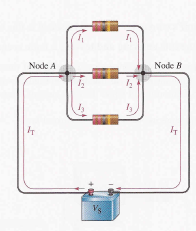# Kirchhoff’s voltage and current law with examples

## What is Kirchhoff’s Voltage Law?

Kirchhoff’s voltage law is a fundamental circuit law that states that the algebraic sum of all the voltages around a closed path is zero or, in other words, the sum of the voltage drops equals the total source voltage.

### Kirchhoff’s loop rule

In an electric circuit, the voltages across the resistors(voltage drops) always have polarities opposite to the source voltage polarity. For example, follow a clockwise loop around the circuit and note that the source polarity is minus-to-plus and each voltage drop is plus-to-minus. Also note that voltage drops across resistors designated as V1, V2, and so on.Also notice that the current is out of the positive side of the source and through the resistors as indicated by the arrows. The current is into the positive side of each resistor and out the negative side. The drop in energy level across a resistor creates a potential difference,or voltage drop, with a plus-to-minus polarity in the direction of the current.

Notice that the voltage from point to point in the circuit equals the source voltage V. Also, the voltage from to is the sum of the series resistor voltage drops. Therefore, the source voltage is equal to the sum of the three voltage drops.This discussion is the example of Kirchhoff’s voltage law, which is generally stated as follow:

The sum of all the voltage around a single closed circuit is equal to the total source voltage in that loop.

### Another Way to State Kirchhoff’s Voltage Law

If all the voltage around a closed path is added and then this total is subtracted from the source voltage, the result is zero. This result occurs because the sum of the voltage drops always equals the source voltage.

The algebraic sum of all voltages (both source and drops) around a closed path is zero.

Therefore, another way of expressing Kirchhoff’s voltage law in equation form is:

V– V– V– V3 – . . . – V = 0

You can verify Kirchhoff’s voltage law by connecting a circuit and measuring each resistor voltage and the source voltage. When the resistor voltage is added together, their sum will equal the source voltage. Any number of resistors can be added.

The following three examples use Kirchhoff’s voltage law to solve circuit problems.### Kirchhoff’s voltage law examples### Examples of kvlWatch also:

## What is Kirchhoff’s Current Law?

You learned Kirchhoff’s voltage law that dealt with voltages in a closed series circuit. Now, you will learn Kirchhoff’s current law that deals with currents in a parallel circuit.

A junction is any point in a circuit where two or more components are connected. So, in a parallel circuit, a junction is where the parallel branches come together. For example, in the circuit, point is one junction and point is another. Let’s start at the positive terminal of the source and follow the current. The total current Ifrom the source is into the junction at point A.

At this point, the current split up among the three branches is indicated. Each of the three branch currents (I1, I2, and I) is out of junction A. Kirchhoff’s current law says that the total current into junction A is equal to the total current out of junction A.

### Kirchoff’s current law formula

II1 + I3Now, following the currents through the three branches, you see that they come back together at point B. Currently, I1, I2, and Iare into junction B, and Iis out of junction B. Kirchhoff’s current law formula at junction B is, therefore, the same as at junction A.

II1 + I3

### General Formula for Kirchhoff’s Current Law

The previous discussion used a specific example to illustrate Kirchhoff’s current law shows a generalized circuit junction where a number of branches are connected to a point in the circuit. Current IN (I) through I IN (n) are into the junction (n can be any number). Current OUT (I) through out (m) are not of the junction (m can be any number, but not necessarily equal to n).

By Kirchhoff’s current law, the sum of the currents into a junction must equal the sum of the currents into a junction must equal the must of the currents out of junction. With reference to Figure, the general formula for Kirchhoff’s current law is:

IN (1)  IN (2) + . . .I IN (n) OUT(1)OUT(2) +  . . . + I OUT(m)### kirchhoff’s current law examplesWatch also:

### One Comment

1. Coleman says:

This excellent website certainly has all of the information and facts I
wanted concerning this subject and didn’t know who to ask.